# PLC Counter | How counters work in PLC? | Basic Guidance

Hey Friends! How are you? I hope you like the tutorial of PLC instructions.

We have seen Bit logic instructions, Arithmetic and Comparator instructions, PLC timer instructions, PLC programming languages in my previous tutorial.

In this blog, we are going to see about the PLC counter instructions with practical PLC counter programming examples.

## PLC Counter | What is counter in PLC?

A PLC Counter is a function in PLC programming that is used to measure things like how many times an event has happened in a process or how many times a product has been produced.

In this blog, we are going to see how plc counters work? and how we can use counters in the PLC program.

### Siemens PLC Counter Block:

In Siemens PLC we have three types of counter functions like,

• Up Counter
• Down Counter
• Up and Down Counter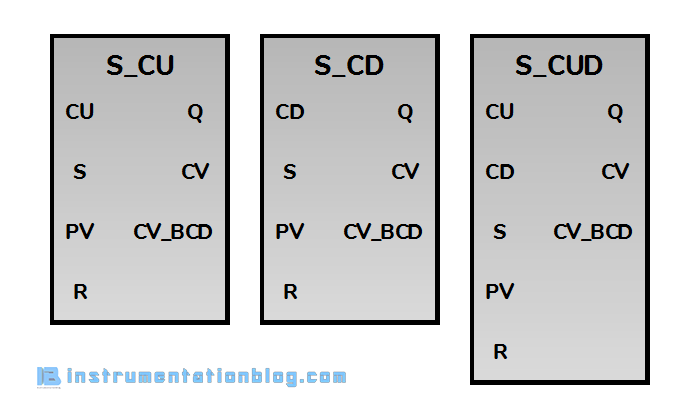These are the blocks that are used as a counter in PLC ladder logic. All counter blocks have some inputs and some outputs.

Inputs,

• CU – Count Up Input
• CD – Count Down Input
• S – Set Input for presetting counter
• PV – Value for presetting counter
• R – Reset Input

Outputs,

• Q – Status of Counter
• CV – Current counter value
• CV_BCD – Current counter value in BCD coded

#### Up Counter(S_CU)For each pulse at Count Up(CU) bit, current Counter Value(CV) will be increased by 1. When there is a pulse at Set input(S), it will set Presetting Value(PV) at the current Counter Value(CV).

When there is a pulse at Reset input(R), the counter block will get reset and the current counter value is set at 0 again.

Example,

There is an indicating alarm when the production limit reaches 100.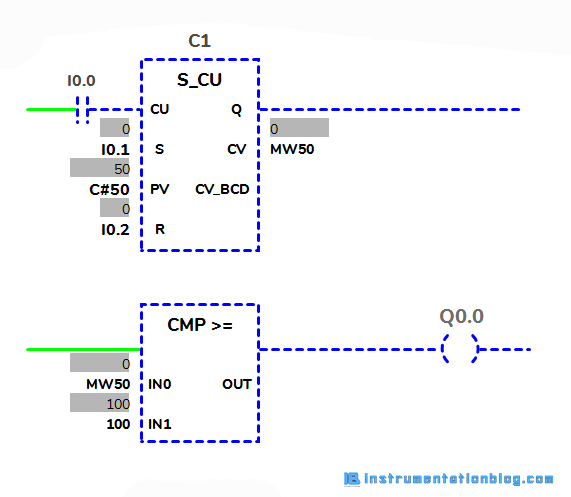#### Down Counter(S_CD)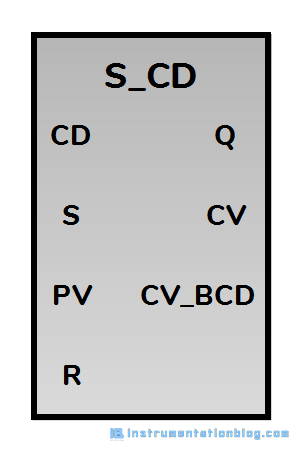For each pulse at Count Down(CD) bit, current Counter Value(CV) will be decreased by 1. When there is a pulse at Set input(S), it will set Presetting Value(PV) at the current Counter Value(CV).

When there is a pulse at Reset input(R), the counter block will get reset and the current counter value is set at 0 again.

Example,

There is an alarm showing that the production limit has reached below 10.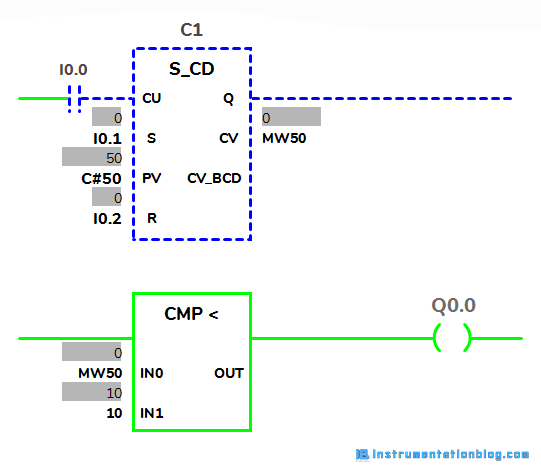#### Up Down Counter(S_CUD)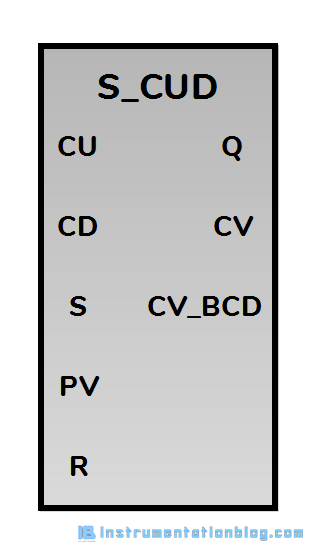This block has both the count up and count down functions.

At each pulse at Count Up(CU) bit, it will increase the current Counter Value(CV) and each pulse at Count Down(CD) bit, will decrease the current Counter Value(CV).

When there is a pulse at the Set input(S), it will set Presetting Value(PV) at the current Counter Value(CV).

When there is a pulse at Reset input(R), the counter block will get reset and the current Counter value is set at 0 again.

Example,

Suppose there is a limit of 50 vehicles in a parking lot. So it will show the vacant positions and parking full indicator.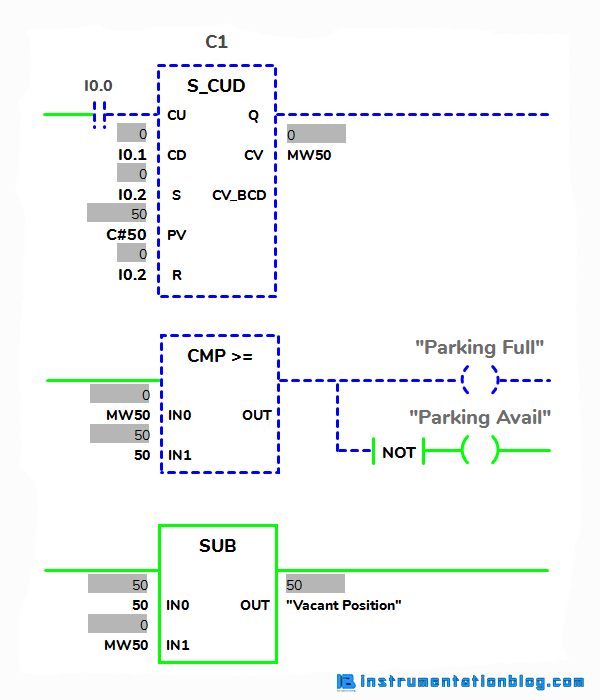### Allen Bradley PLC Counter Block:

In Allen Bradley PLC we have three types of counter functions like,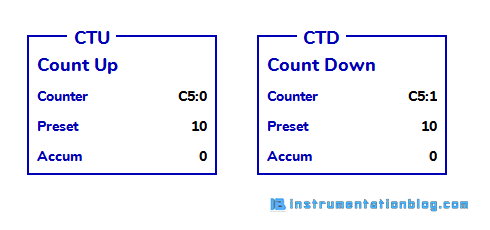The inputs and outputs of these counter blocks have some inputs and outputs.

Inputs,

• CU – Count Up Input
• CD – Count Down Input
• Preset – Value for presetting counter

Outputs,

• Accum – Current counter value

#### Counter Up(CTU)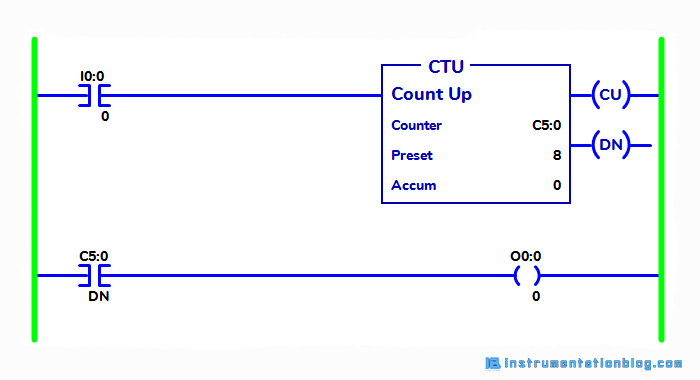This instruction is used to count up the value. Whenever there is a pulse at the input of the CTU block, it will increase the accumulator value by 1.

When the accumulator value is equal to or greater than the preset value, the DN bit is set. The DN bit is true until the accumulator value goes below the preset value or CTU is reset using RES instruction.

#### Counter Down(CTD)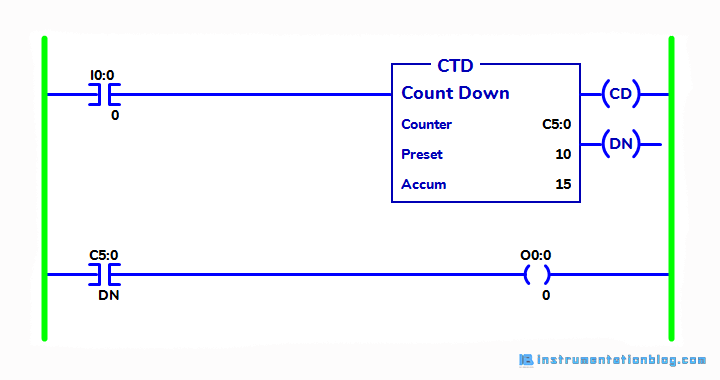This instruction is used to count down the value. Whenever there is a pulse at the input of the CTD block, it will decrease the accumulator value by 1.

When the accumulator value is equal to or greater than the preset value, the DN bit is set. The DN bit is true until the accumulator value goes below the preset value.

### PLC Counter Applications:

• Bottle Filling Plant
• Parking Space Application
• Sequential Controller Application

### Summary(PLC Counter Instructions)

This is all the information about the counter function in PLC programming. I hope you like this blog, if you want more tutorial about PLC programming then please comment down below.

Programmable Logic Controller (PLC) related more articles ⇒

Your feedback is valuable to us. Thank you.

1.